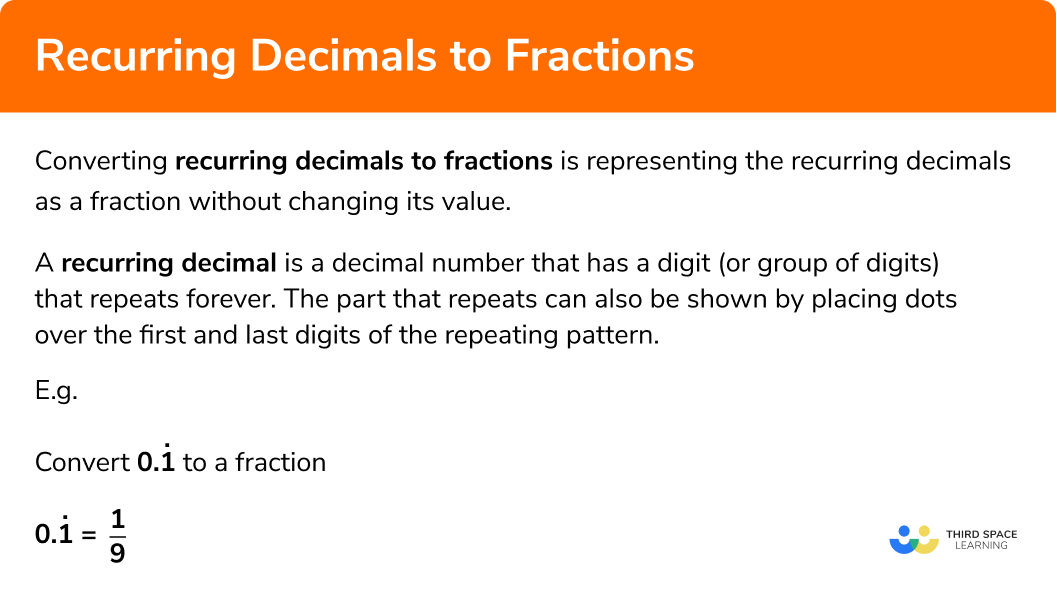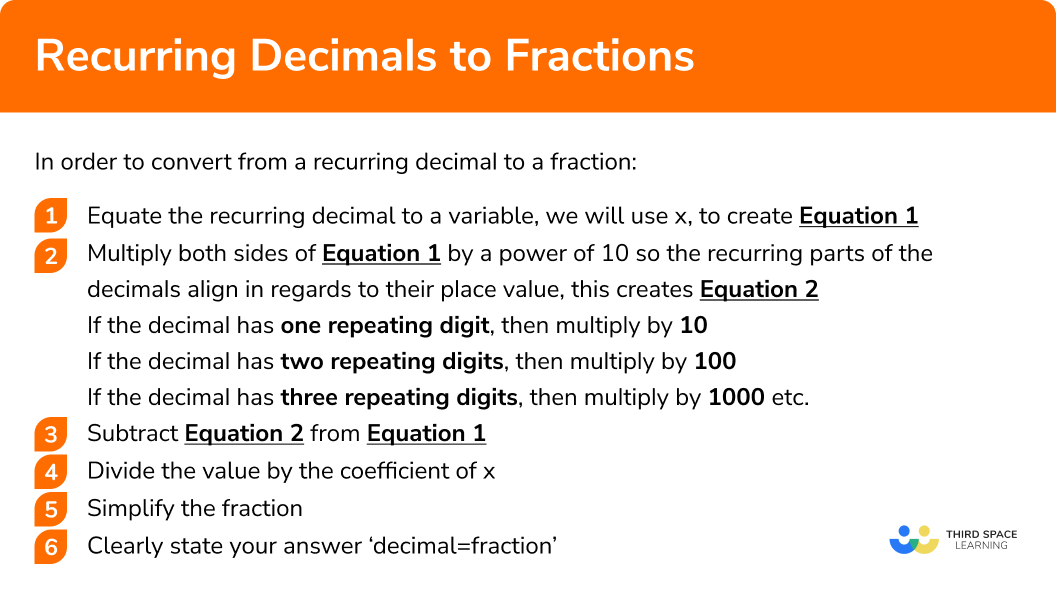GCSE Maths Number FDP Converting FDP

Recurring Decimals To Fractions

# Converting Recurring Decimals To Fractions

Here we will learn about converting recurring decimals to fractions including how to define a recurring decimal.

There are also converting recurring decimals to fractions worksheets based on Edexcel, AQA and OCR exam questions, along with further guidance on where to go next if you’re still stuck.

## What is converting recurring decimals to fractions?

Converting recurring decimals to fractions is representing a recurring decimal as a fraction without changing its value.

A recurring decimal is a decimal number that has a digit (or group of digits) that repeats forever. The part that repeats can also be shown by placing dots over the first and last digits of the repeating pattern.

E.g.

\begin{aligned} &0 . \dot{3} = 0.333333… \\\\ &0 . \dot{2}\dot{4} = 0.24242424… \\\\ &0 . \dot{1}\dot{2}\dot{3} = 0.123123123… \end{aligned}

are all recurring decimals

### What is converting recurring decimals to fractions?Note: these are sometimes called repeating decimals

## How to convert recurring decimals to fractions

In order to convert from a recurring decimal to a fraction:

1Equate the recurring decimal to a variable, we will use x, to create Equation 1

2Multiply both sides of Equation 1 by a power of 10 so the recurring parts of the decimals align in regards to their place value, this creates Equation 2

• If the decimal has one repeating digit, then multiply by 10
• If the decimal has two repeating digits, then multiply by 100
• If the decimal has three repeating digits, then multiply by 1000 etc.

3Subtract Equation 2 from Equation 1

4Divide the value by the coefficient of x

5Simplify the fraction

### Explain how to convert from a recurring decimal to a fraction in 6 steps## Recurring decimals to fractions examples

### Example 1: converting with a simple recurring decimal (in the tenths column only)

Convert 0.\dot{1} to a fraction

1. Equate the recurring decimal to a variable, we will use x, to create Equation 1

$0 . \dot{1}=x \hspace{3cm} \text{Equation 1}$

2Multiply both sides of Equation 1 by a power of 10 so the recurring element of the decimals align (this creates Equation 2)

You now need to multiply 0 . \dot{1} by a power of 10 (e.g 10,100,1000) so the recurring elements of the decimal align in regards to their place value.

Because 0 . \dot{1} has one repeating digit, we will multiply by 10

$0 . \dot{1}\times10=1.\dot{1}$

Remember because you are multiplying the whole of Equation 1 you also need to multiply the variable x by 10 (see below)

\begin{aligned} 0 . \dot{1}&=x \hspace{3cm} \text{Equation 1} \\\\ 0 . \dot{1} \times 10&=x \times 10 \\\\ 1.\dot{1} &=10 x \hspace{2.5cm} \text{Equation 2} \end{aligned}

3Subtract Equation 2 from Equation 1

\begin{aligned} 1 . \dot{1}&=10x \hspace{3cm} \text{Equation 2}\\\\ 0. \dot{1}&=x \hspace{3.5cm} \text{Equation 1} \\\\ 1&=9 x \end{aligned}

4Divide the value by the coefficient of x

\begin{aligned} 1&=9x\\\\ \frac{1}{9}&=x \end{aligned}

We originally stated that x was equal to 0 .\dot{1}

If x is equal to both 0 .\dot{1}. and \frac{1}{9} we can state that 0 .\dot{1} and \frac{1}{9} are equal to one another

5Simplify the fraction

This fraction cannot be simplified as the only factor that 1 and 9 share is 1

$0 . \dot{1}= \frac{1}{9}$

### Example 2: converting with a recurring decimal with simplifying

Convert 0 .\dot{1}\dot{2} to a fraction

Equate the recurring decimal to a variable to create Equation 1

Multiply both sides of Equation 1 by a power of 10 so the recurring element of the decimals align (this creates Equation 2 )

Subtract Equation 2 from Equation 1

Divide the value by the coefficient of x

Simplify the fraction

### Example 3: converting with a recurring decimal (starting in the hundredths column only)

Convert 0.0\dot{1} to a fraction

Equate the recurring decimal to a variable to create Equation 1

Multiply both sides of Equation 1 by a power of 10 to create Equation 2

Subtract Equation 2 from Equation 1

Divide the value by the coefficient of x

Simplify the fraction

### Example 4: converting with a recurring decimal (in the tenths and hundredths column)

Convert 0.\dot{2}\dot{3} to a fraction

Equate the recurring decimal to a variable to create Equation 1

Multiply both sides of Equation 1 by a power of 10 to create Equation 2

Subtract Equation 2 from Equation 1

Divide the value by the coefficient of x

Simplify the fraction

### Example 5: converting with a recurring decimal greater than 1

Convert 8.\dot{7} to a fraction

Equate the recurring decimal to a variable to create Equation 1

Multiply both sides of Equation 1 by a power of 10 to create Equation 2

Subtract Equation 2 from Equation 1

Divide the value by the coefficient of x

Simplify the fraction

### Example 6: converting with a more complex recurring decimal

Convert 4.0\dot{4}\dot{6} to a fraction

Equate the recurring decimal to a variable to create Equation 1

Multiply both sides of Equation 1 by a power of 10  to create Equation 2

Subtract Equation 2 from Equation 1

Divide the value by the coefficient of x

Simplify the fraction

### Common misconceptions

• Multiplying by a power of 10

You must make sure you are multiplying by the correct power of 10 (e.g 10, 100, 1000) so the recurring aspect of decimal can be eliminated.

E.g
Multiplying 0 . \dot{1}\dot{3} by 10 does not help us eliminate the recurring decimal by subtraction (in step 3). In this example you need to multiply by 100 because you can now eliminate the recurring aspect of the decimal by subtraction.

Incorrect

$0 . \dot{1}\dot{3} \times 10 = 1.\dot{3}\dot{1}$

1 . \dot{3}\dot{1}-0 . \dot{1}\dot{3} does not eliminate the recurring decimal

Correct

$0 . \dot{1}\dot{3} \times 100 = 13.\dot{1}\dot{3}$

13.\dot{1}\dot{3} - 0 . \dot{1}\dot{3} does eliminate the recurring decimal

• Fractions with a decimals

Fractions should not include a decimal

E.g
\frac{0.1}{2}

In this example you can multiply the numerator and denominator by 10 to be left with  \frac{1}{20}

• Not simplifying the fraction

Always check to see if you have simplified the fraction into its simplest form

Recurring decimals to fractions is part of our series of lessons to support revision on comparing fractions, decimals and percentages. You may find it helpful to start with the main comparing fractions, decimals and percentages lesson for a summary of what to expect, or use the step by step guides below for further detail on individual topics. Other lessons in this series include:

### Practice converting recurring decimals to fractions questions

1. What should you multiply 0 . \dot{2} by to help to eliminate the recurring decimal?

110100It does not matter0 . \dot{2}\times10=2 . \dot{2}

which allows you to eliminate the recurring decimal

2. What should you multiply 0 . \dot{1}\dot{4} by to help to eliminate the recurring decimal?

110100It does not matter0 . \dot{1}\dot{4}\times100=14.\dot{1}\dot{4}

which allows you to eliminate the recurring decimal

3. What should you multiply 0 .0 \dot{1}\dot{5} by to help to eliminate the recurring decimal?

110100It does not matter0 .0 \dot{1}\dot{5}\times100=1.5 \dot{1}\dot{5}

which allows you to eliminate the recurring decimal

4. What is 0. \dot{2} as a fraction?

\frac{2}{9}\frac{2}{10}\frac{2}{99}\frac{2}{999}Equate to x to create Equation 1

Multiply the recurring decimal by 10 to create Equation 2

Eliminate the recurring decimal by subtracting the equations

Divide by 9 (cannot be simplified)

5. What is 0. \dot{0}\dot{2} as a fraction?

\frac{2}{9}\frac{2}{100}\frac{2}{99}\frac{2}{999}Equate to x to create Equation 1

Multiply the recurring decimal by 100 to create Equation 2

Eliminate the recurring decimal by subtracting the equations

Divide by 99 (cannot be simplified)

6. What is 0. \dot{0}\dot{0}\dot{2}  as a fraction?

\frac{2}{9}\frac{2}{1000}\frac{2}{99}\frac{2}{999}Equate to x to create Equation 1

Multiply the recurring decimal by 1000 to create Equation 2

Eliminate the recurring decimal by subtracting the equations

Divide by 999 (cannot be simplified)

### Converting recurring decimals to fractions GCSE questions

1. Convert 0.\dot{2}\dot{7} to a fraction, give your answer in its simplest form

(4 Marks)

Multiping 0.\dot{2}\dot{7} by 100 or 27.\dot{2}\dot{7}

(1)

Subtracting equations to create 27=99x

(1)

\frac{27}{99}

(1)

\frac{3}{11}

(1)

2. Convert 0.\dot{0}\dot{7} to a fraction

(3 Marks)

Multiping 0.\dot{0}\dot{7} by 100 or 7.\dot{0}\dot{7}

(1)

Subtracting equations to create 7=99x

(1)

\frac{7}{99}

(1)

3. Prove 0.\dot{3}\dot{9} =\frac{13}{33}

(4 Marks)

Multiping 0.\dot{3}\dot{9} by 100 or 39.\dot{3}\dot{9}

(1)

Subtracting equations to create 39=99x

(1)

\frac{39}{99}

(1)

\frac{39}{99}=\frac{13}{33}

(1)

4. Prove 0.1\dot{2}\dot{6} =\frac{25}{198}

(4 Marks)

Multiping 0.1\dot{2}\dot{6} by 100 or 12.6\dot{2}\dot{6}

(1)

Subtracting equations to create 12.5=99x

(1)

\frac{125}{990}

(1)

\frac{125}{990}=\frac{25}{198}

(1)

## Learning checklist

You have now learned how to:

• Convert a recurring decimal to a fraction

## Still stuck?

Prepare your KS4 students for maths GCSEs success with Third Space Learning. Weekly online one to one GCSE maths revision lessons delivered by expert maths tutors.

Find out more about our GCSE maths tuition programme.